2018-05-23 12:01:20 qq_25011449 阅读数 458

# 李宏毅深度学习_Backpropagation

## Background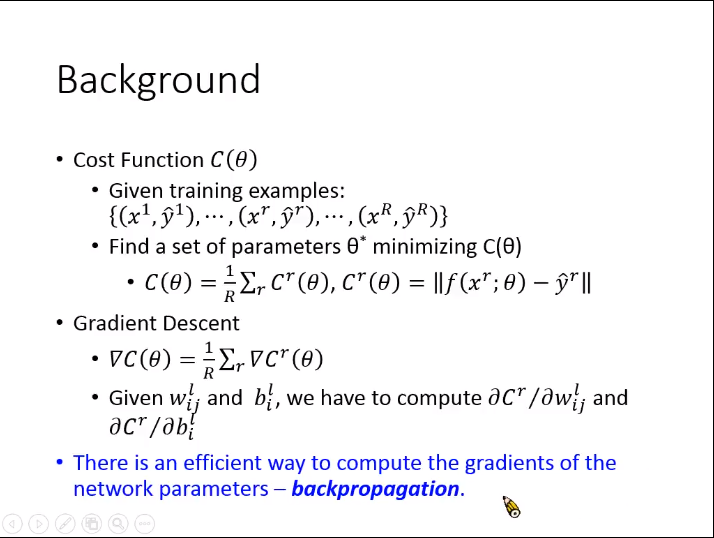## 链式法则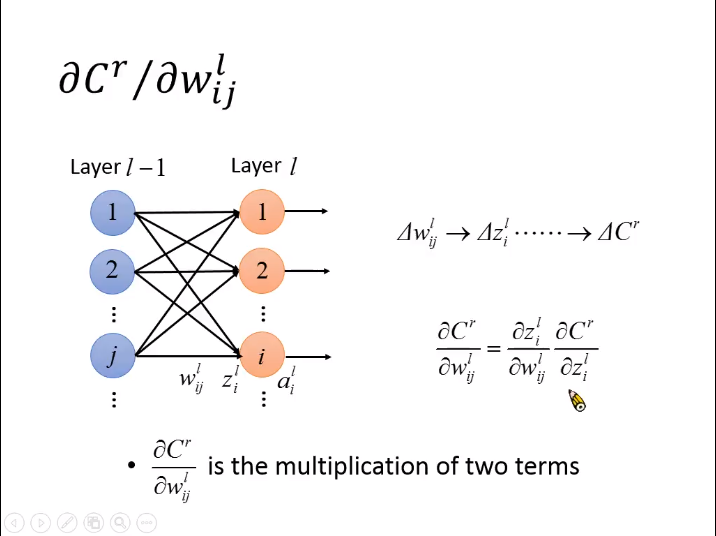## 计算链式法则中拆解的两部分

1. 计算$\mathrm{\partial }{z}_{i}^{l}/\mathrm{\partial }{w}_{ij}^{l}$$\partial z^l_i/ \partial w^l_{ij}$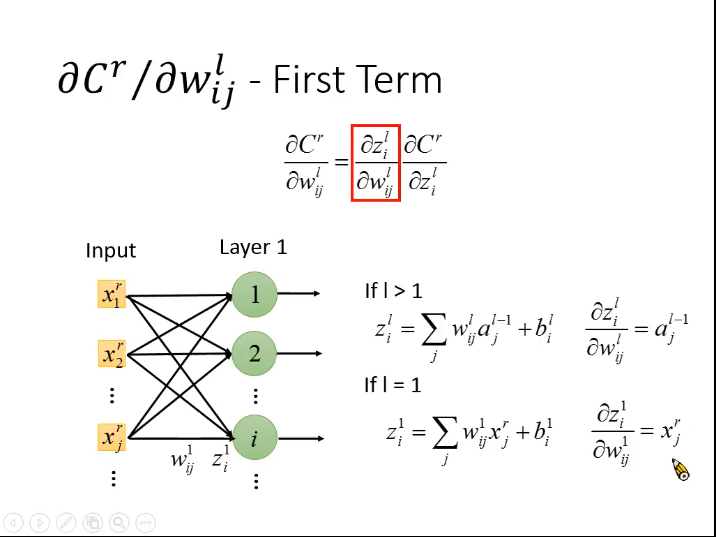• $l>1$$l>1$。即当权值不是第一层时，导数为${a}_{j}^{l-1}$$a^{l-1}_j$
• $l=1$$l=1$。即权值为第一层时，导数为${x}_{j}^{r}$$x^r_j$

2. 计算$\mathrm{\partial }{C}^{r}/\mathrm{\partial }{z}_{i}^{l}$$\partial C^r/ \partial z^l_{i}$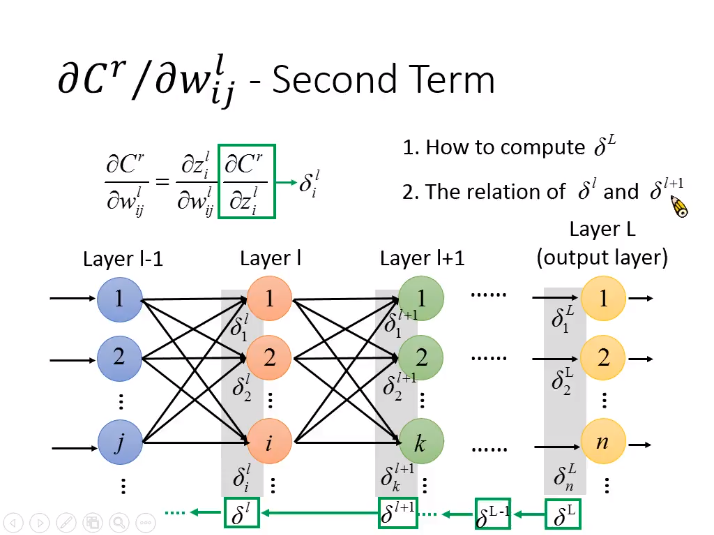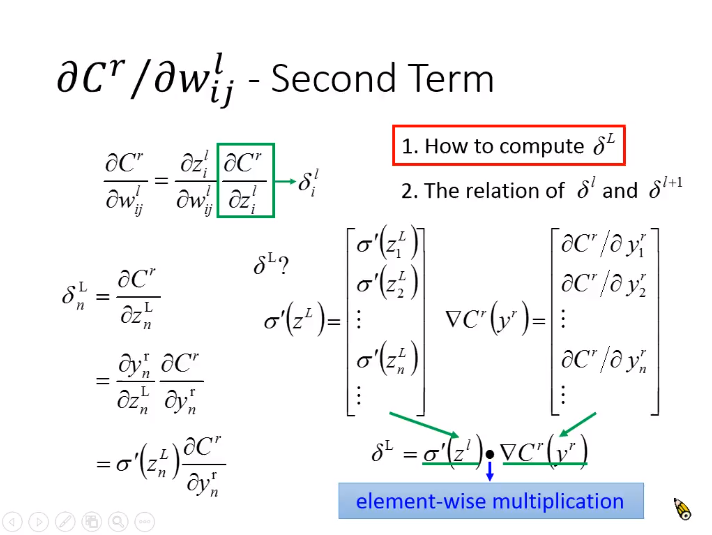${\delta }_{n}^{L}={\sigma }^{\prime }\left({z}_{n}^{L}\right)\frac{\mathrm{\partial }{C}^{r}}{\mathrm{\partial }{y}_{n}^{r}}$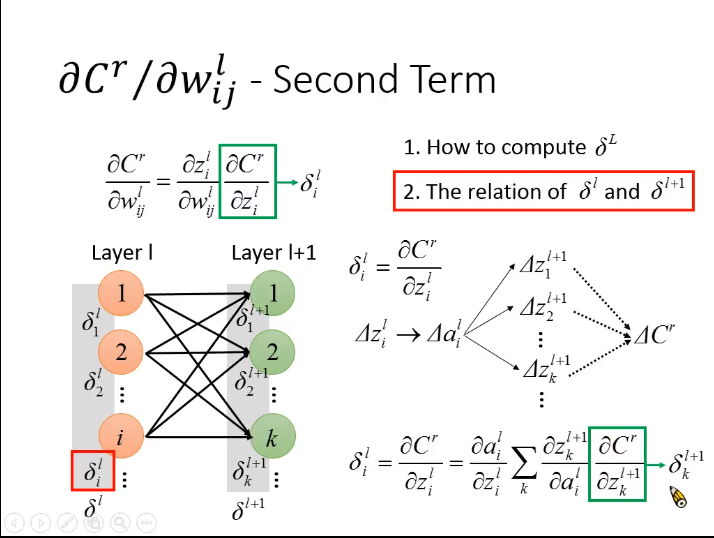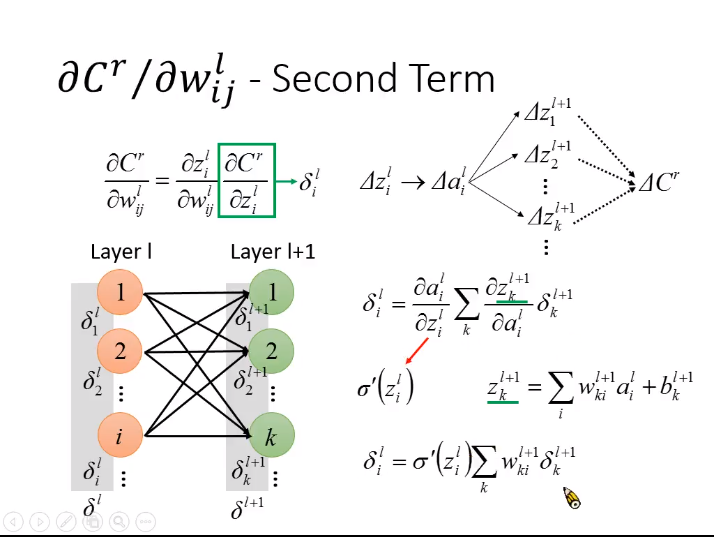${z}_{i}^{l}$$z^l_{i}$的变化$\mathrm{\Delta }{z}_{i}^{l}$$\Delta z^l_{i}$会对${a}_{i}^{l}$$a^l_{i}$造成影响进而影响到下一层的${z}^{l+1}$$z^{l+1}$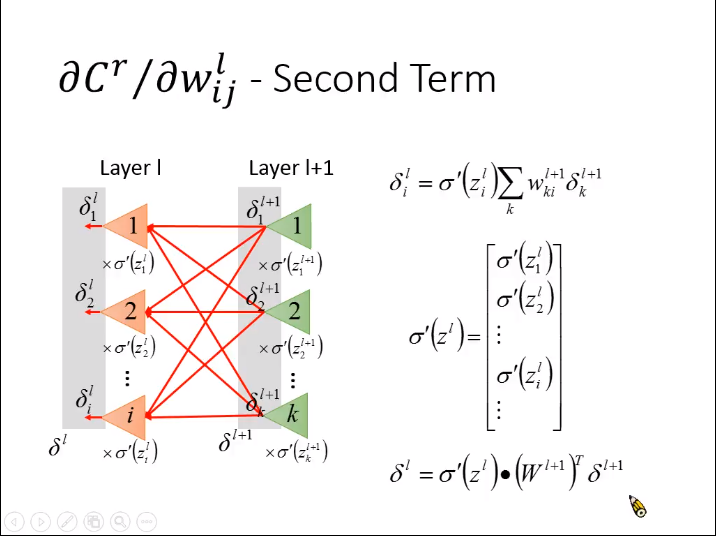${\delta }^{l}={\sigma }^{\prime }\left({z}^{l}\right)\cdot \left({W}^{l+1}{\right)}^{T}{\delta }^{l+1}。$

## 总结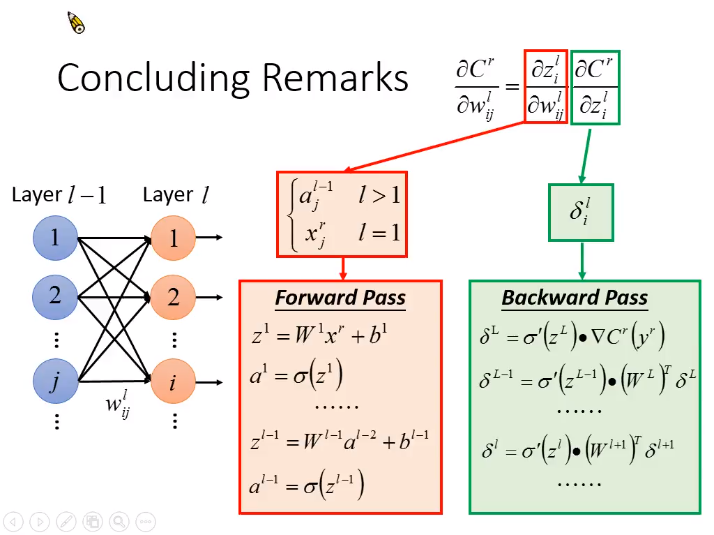2017-06-06 23:00:49 soulmeetliang 阅读数 5536

[机器学习入门] 李宏毅机器学习笔记-10 （Tips for Deep Learning；深度学习小贴士）

PDF VIDEO

# Recipe of Deep Learning# 在 training data上的performance不好## Deeper usually does not imply better## ReLU（Rectified Linear Unit）### ReLU - variant**那么除了ReLU有没有别的activation function了呢？

ReLU is a special cases of Maxout

## Maxout### ReLU is a special cases of Maxout### More than ReLU### Maxout - Training### RMSProp## Hard to find optimal network parameters# 在testing data上performance不好## Early Stopping## Regularization### Regularization - Weight Decay## Dropout### Dropout- Intuitive Reason### Dropout is a kind of ensemble2019-04-01 21:10:15 YDC123458 阅读数 689

## Early Stopping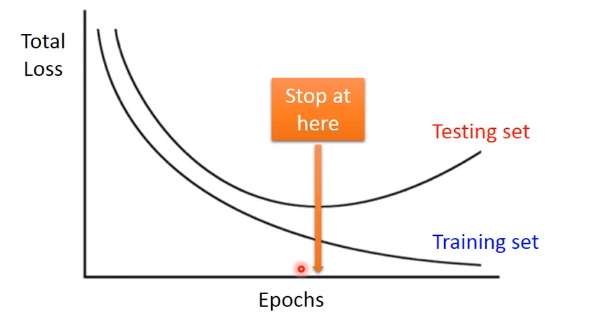## Regularization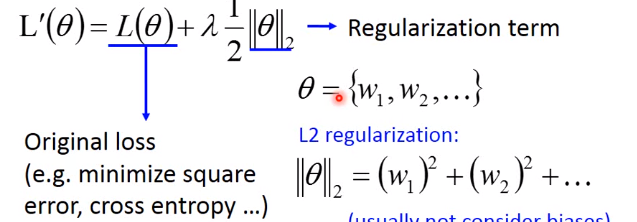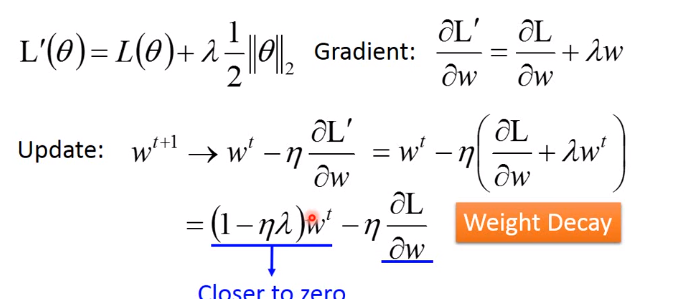都是很小的值，因此每次update之前就相当于乘上一个接近1的数，比如0.99，这相当于前面越来越接近0，相当于在做weight decay。但是深度学习中正则化（Regularization）一般并没有SVM那样明显有帮助。正则化与early stopping所做的事是比较接近的，都是使参数不要离零太远。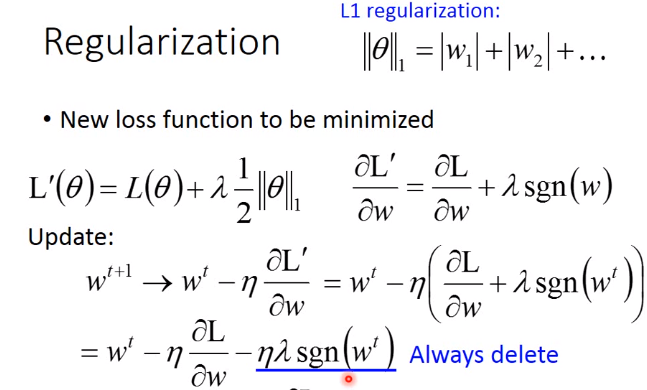每一次update参数时，如果w为正，就是减去一个值让参数变大。如果w是特别大的正值，对于L2正则化减去的也很大，但对于L1减去的都差不多，因此L1可能会保留很多很大的值。如果考虑很小的值比如0.1，0.001，L2下降的就会很慢，因此会保留很多很小的值，而L1减去的值都是固定的值（+1或-1），因此L1的结果比较稀疏。

## Dropout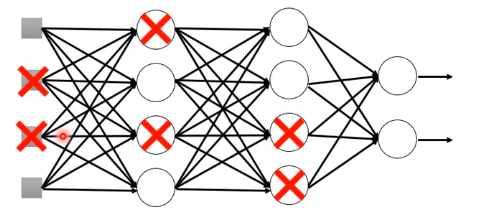training阶段每一个神经元被丢掉的概率都是p%,每一次update前都进行上图所示的dropout。 在testing时，不进行dropout，如果训练的dropout是p%，那么测试时weights都会乘上（1-p)%，这些不需要自己操作，pytorch、tensorflow等工具都已经做好了。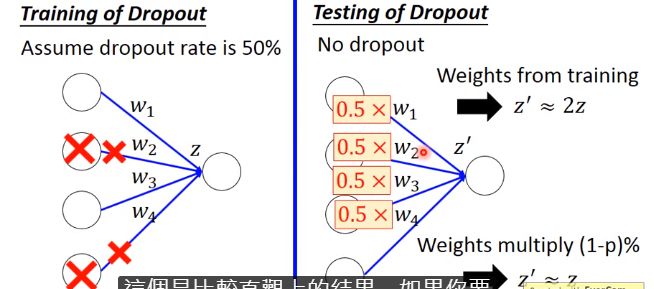上图所示，如果不乘一个值，那么就会不匹配z' = 2z，而都乘0.5时就基本相同了。

其实dropout也相当于一种ensemble方法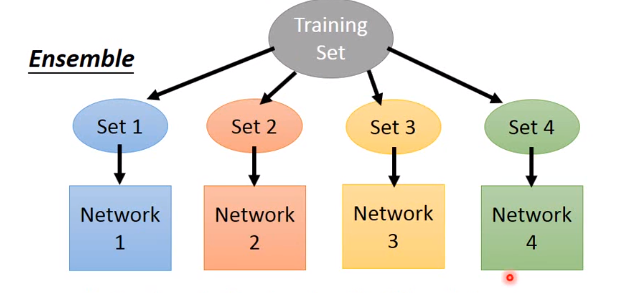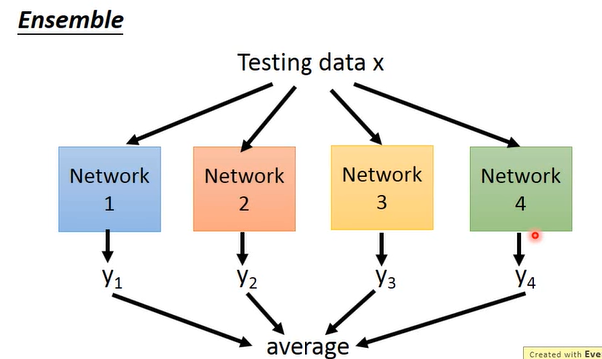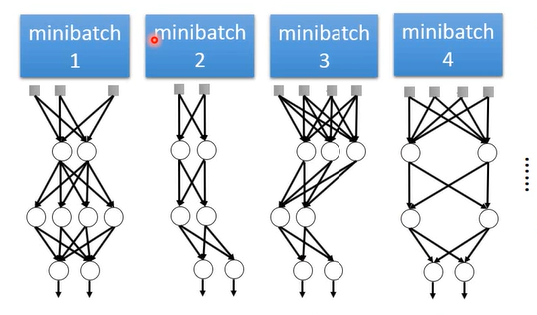注意：每层的weight是共享的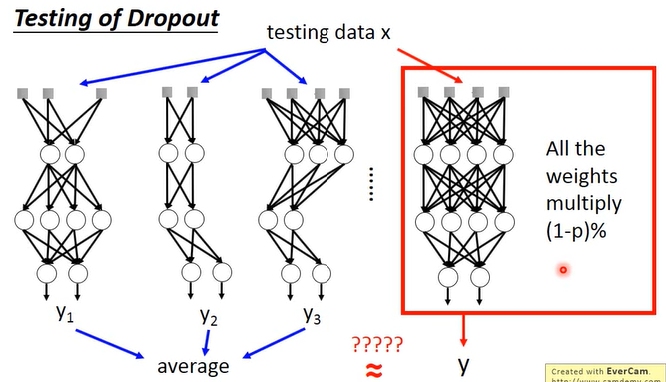ensmeble思想如上图，将所有networks每个都丢一个minibatch然后输出结果进行平均，但上述实际运算太复杂，而神奇的是做测试时不做dropout，而给整个网络乘上（1-p）%效果是和ensmeble完全一样的。

## New activation function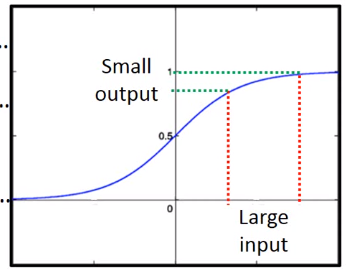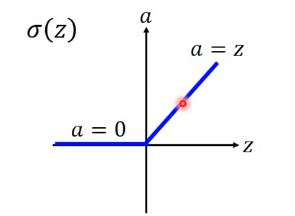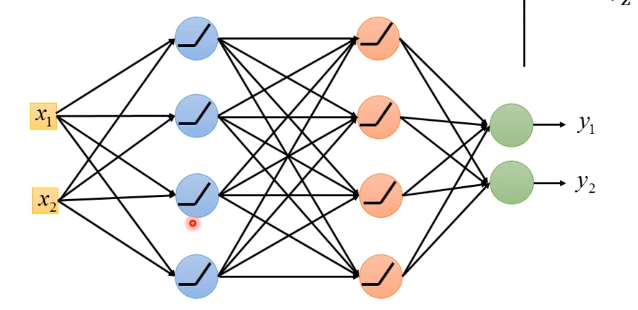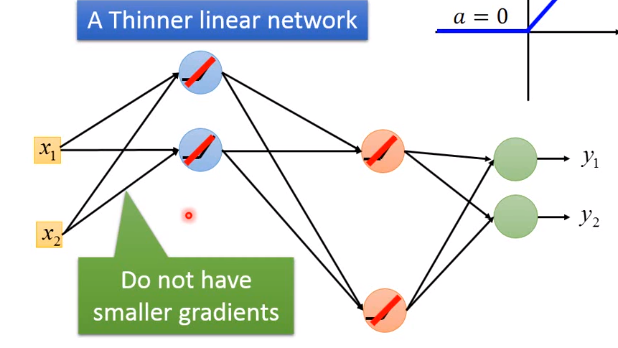ReLU的变体：Leaky ReLU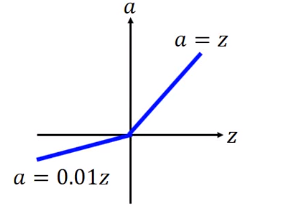ReLU的变体：Parametric ReLU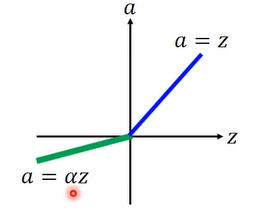Maxout(ReLU是Maxout的一种特殊情况)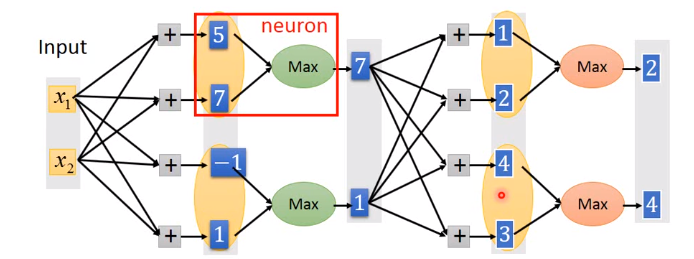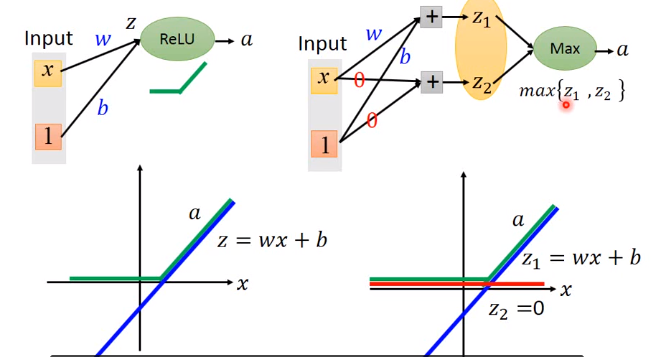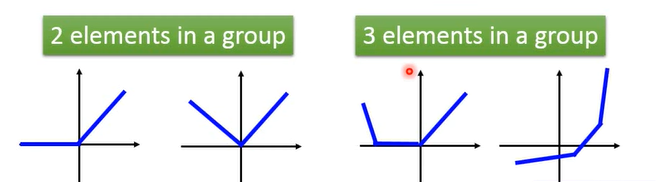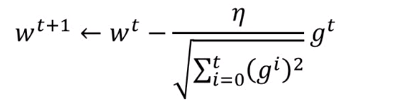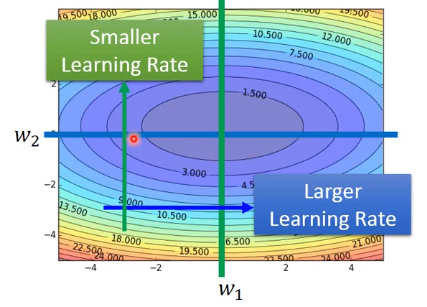RMSProp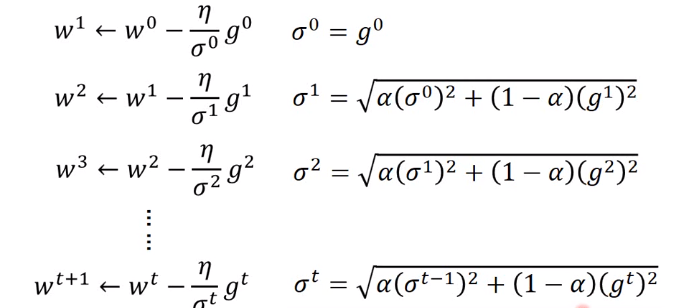Momentum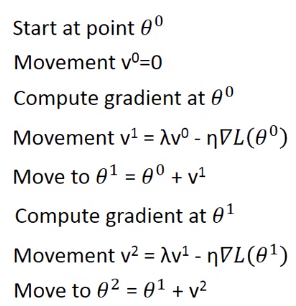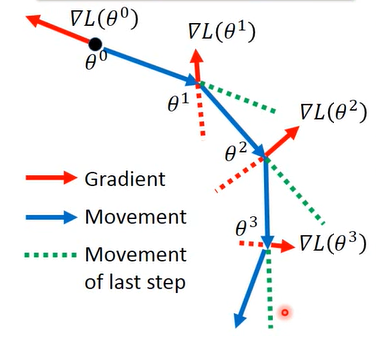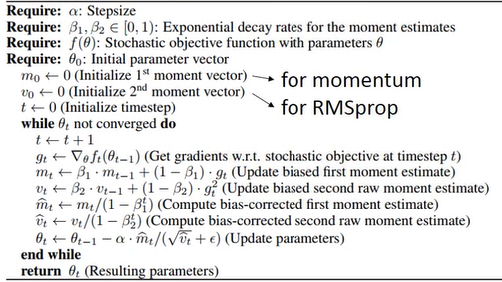------------------------------------------------------------------------------------------------------------------------

2019年4月2日   沙坡村职业技术学院

2018-08-29 09:31:18 Jimmyzqb 阅读数 1995

# 误差分析

## 模型误差原因分析

1. 如果模型在训练集的准确率就不好，说明模型欠拟合，应该重新调整模型，提高训练集的拟合效果；
2. 如果模型在训练集的准确率高而在测试集的准确率很低，就说明了模型存在过拟合的问题。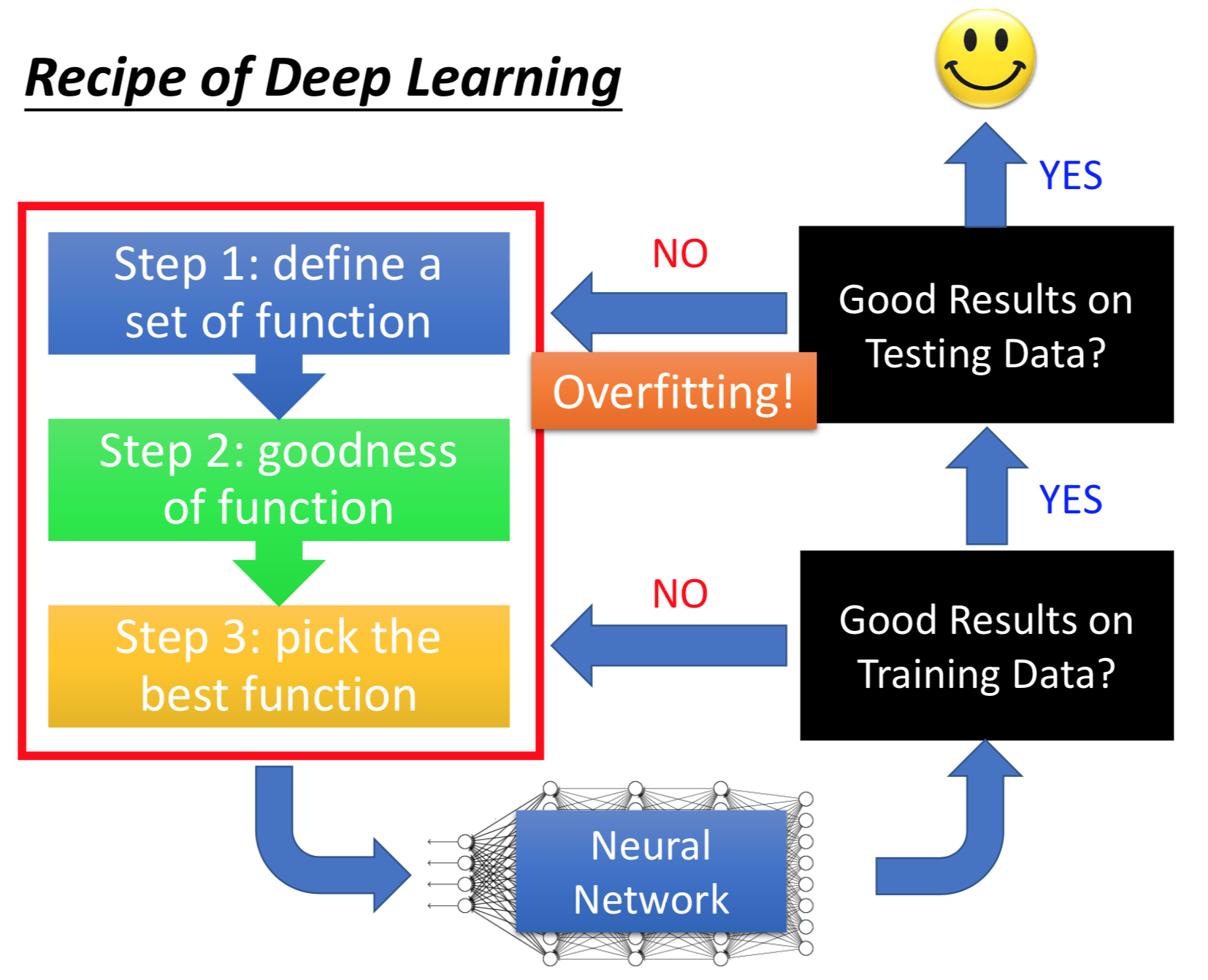$\phantom{\rule{1em}{0ex}}$$\quad$

## 模型优化方案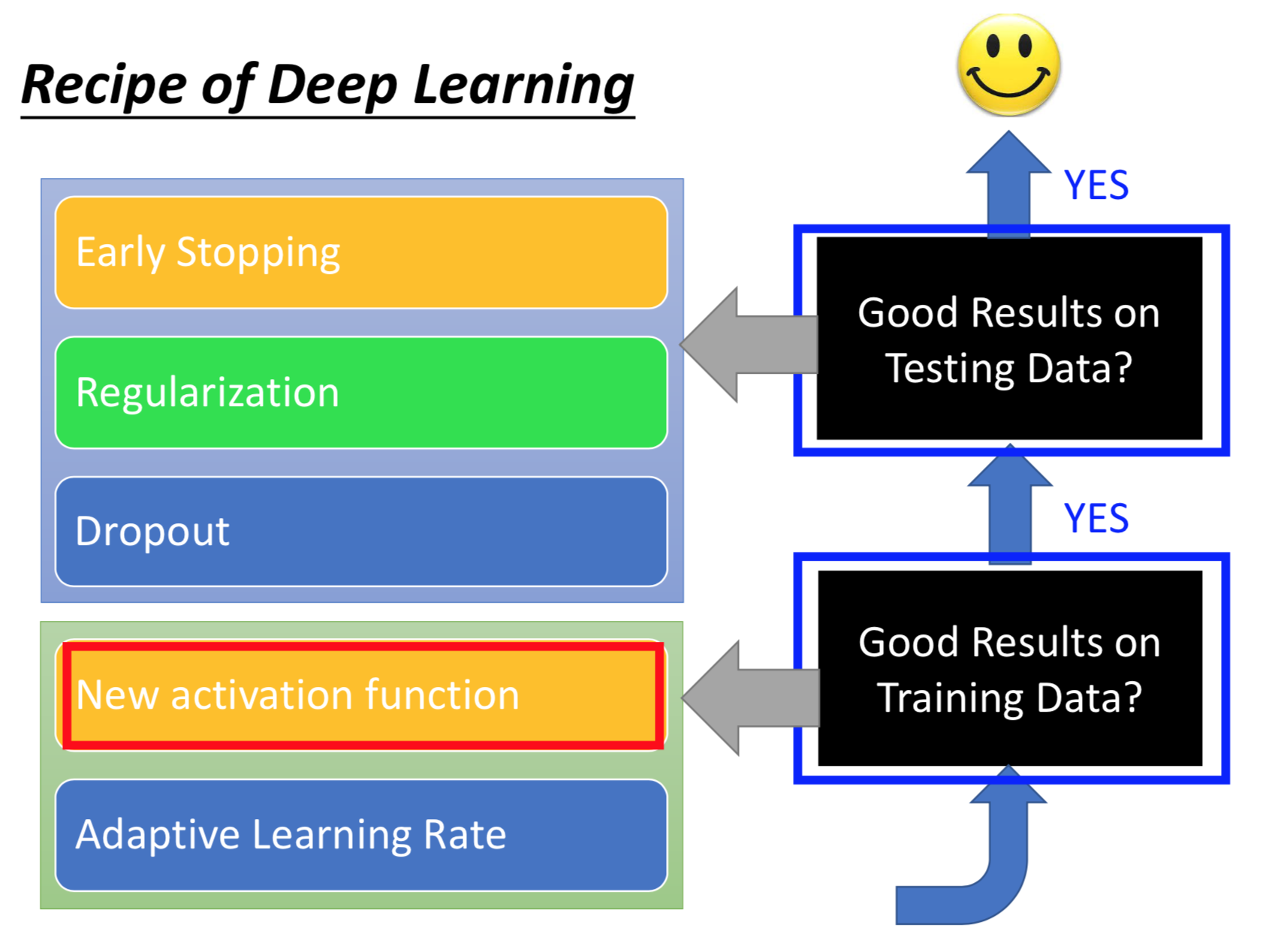$\phantom{\rule{1em}{0ex}}$$\quad$

# New activation function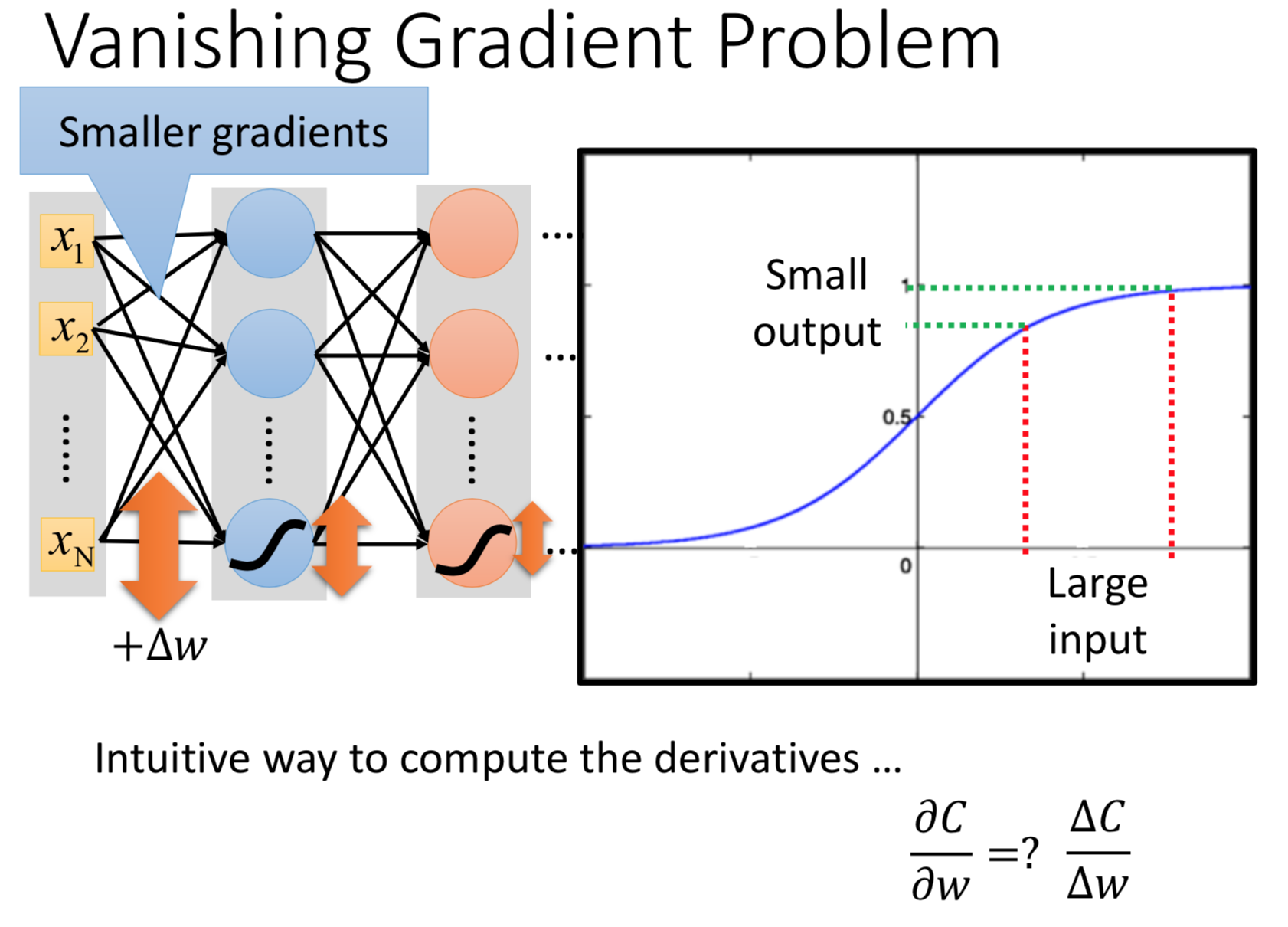$\phantom{\rule{1em}{0ex}}$$\quad$

## ReLU

ReLU全称Rectified Linear Unit，以ReLU作为激活函数可以解决梯度消失问题，通过ReLU对于一个样本通常整个神经网络被简化为较简单的形式（剔除为output为0的神经元）。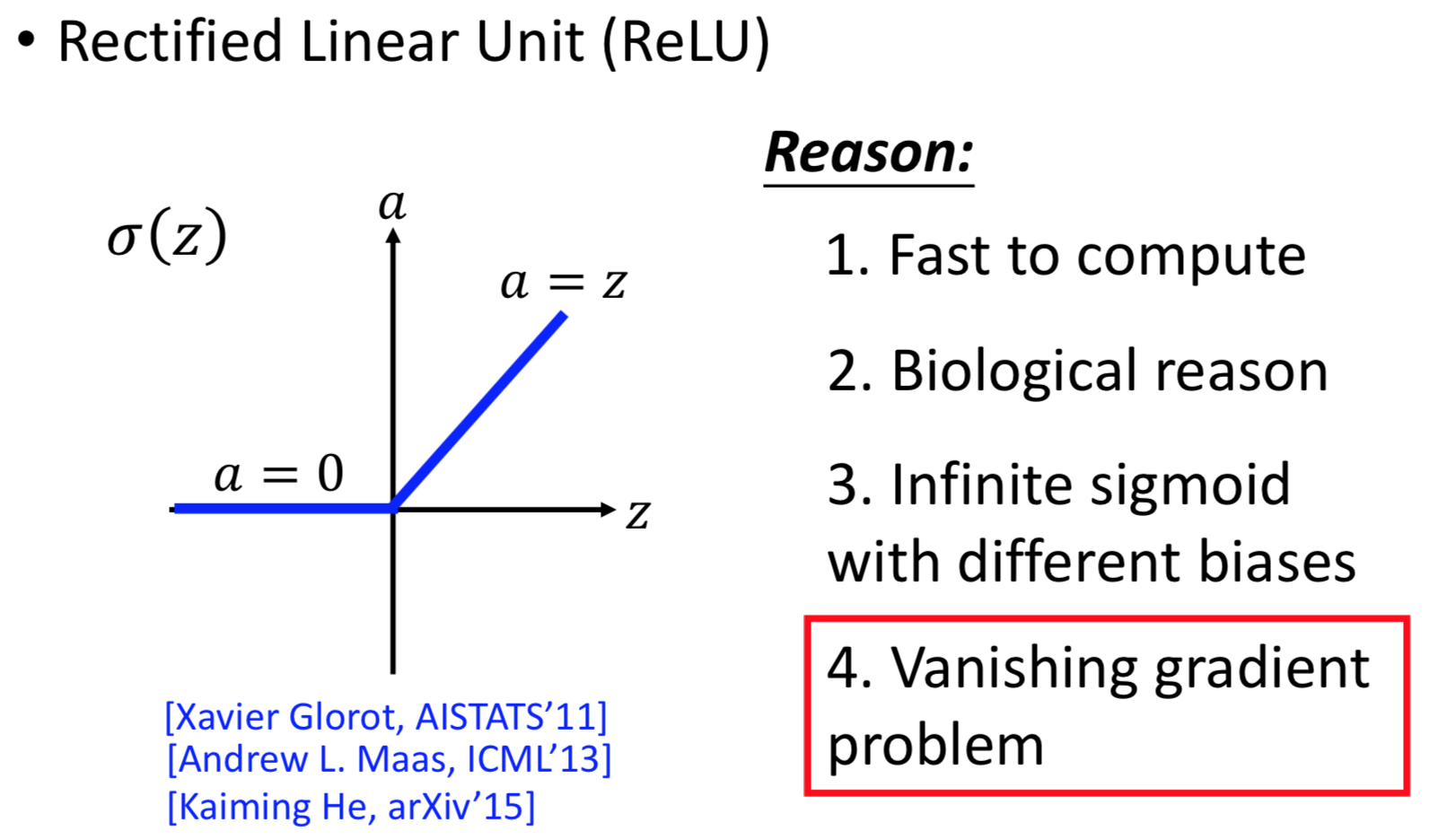ReLU还有多种变形：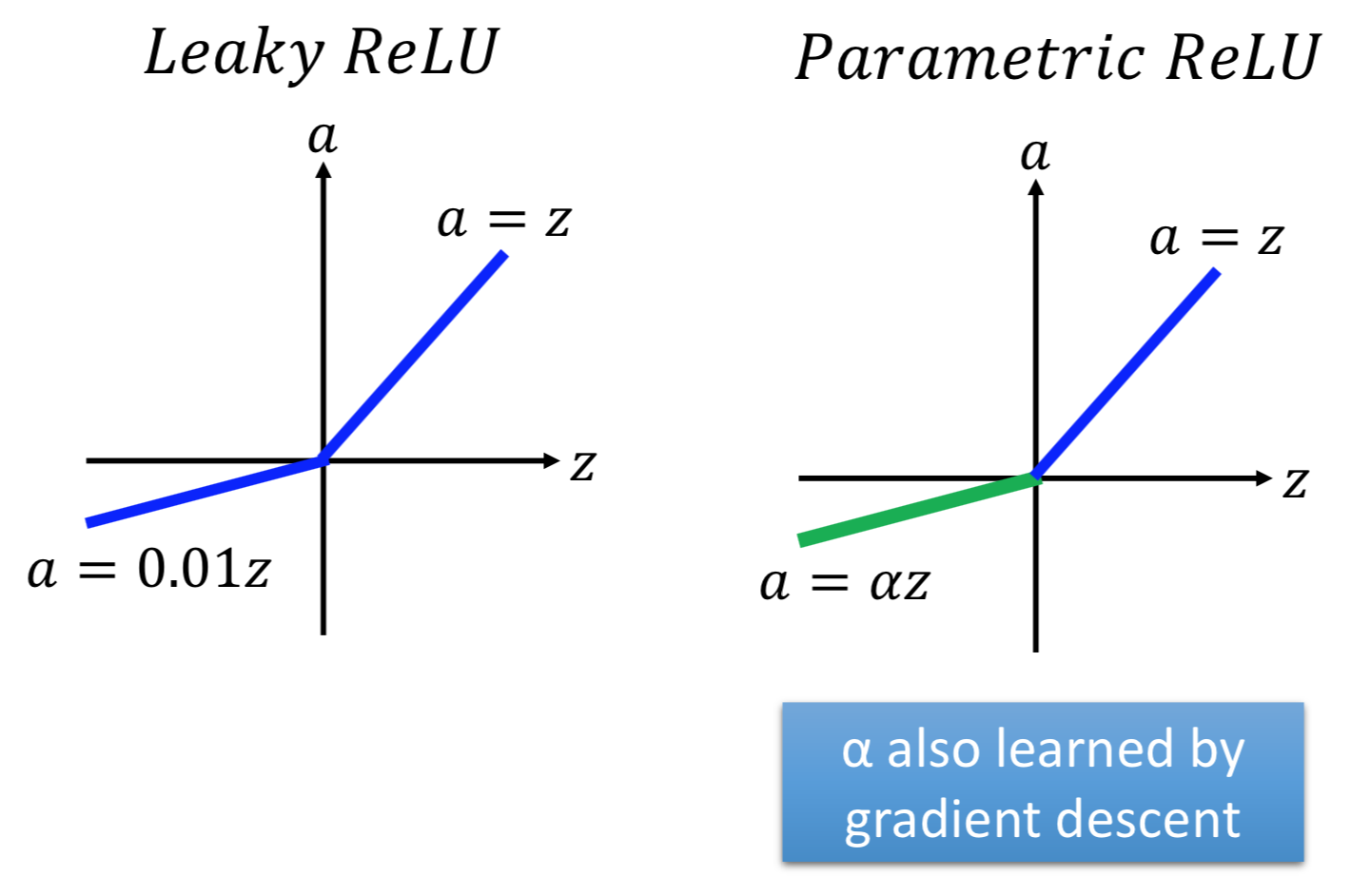$\phantom{\rule{1em}{0ex}}$$\quad$

## Maxout

### Maxout介绍

Maxout让神经网络自动地学习适合的激活函数，如图，$\left({x}_{1},{x}_{2}\right)$$(x_1,x_2)$作为输入向量，连接4个神经元，得到4个数值，以两个为一组，取组内最大值，再重复一次得到2、4。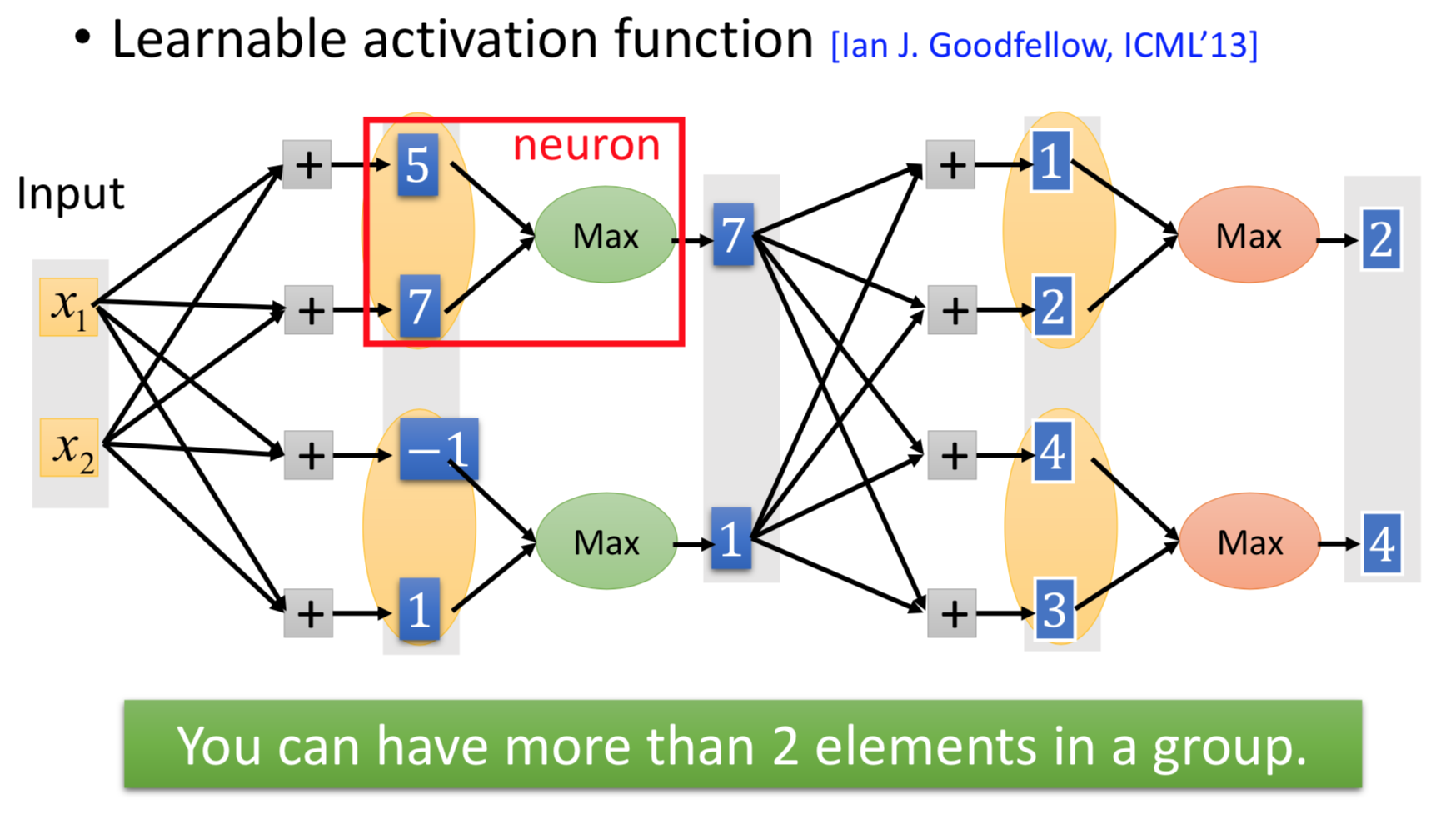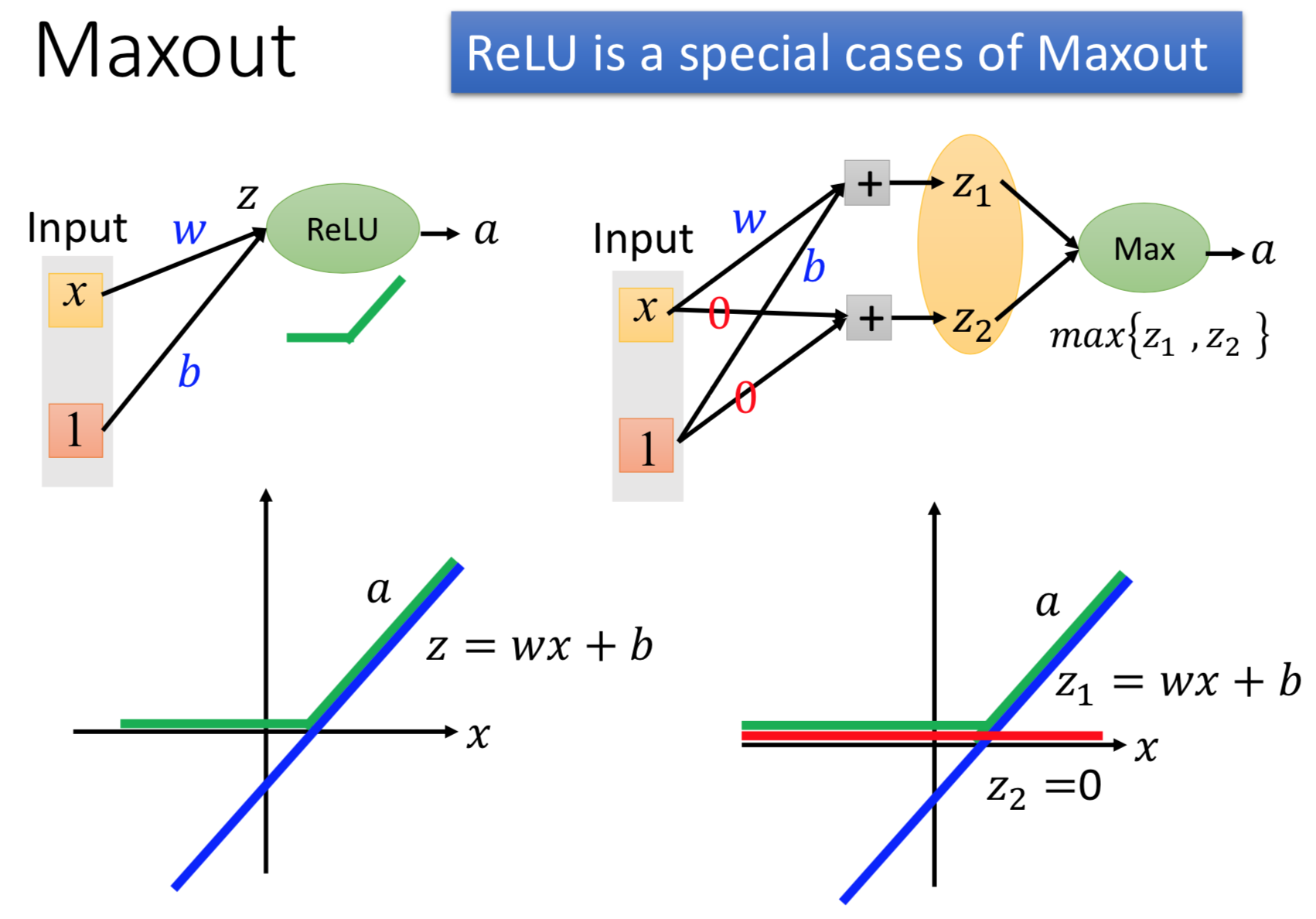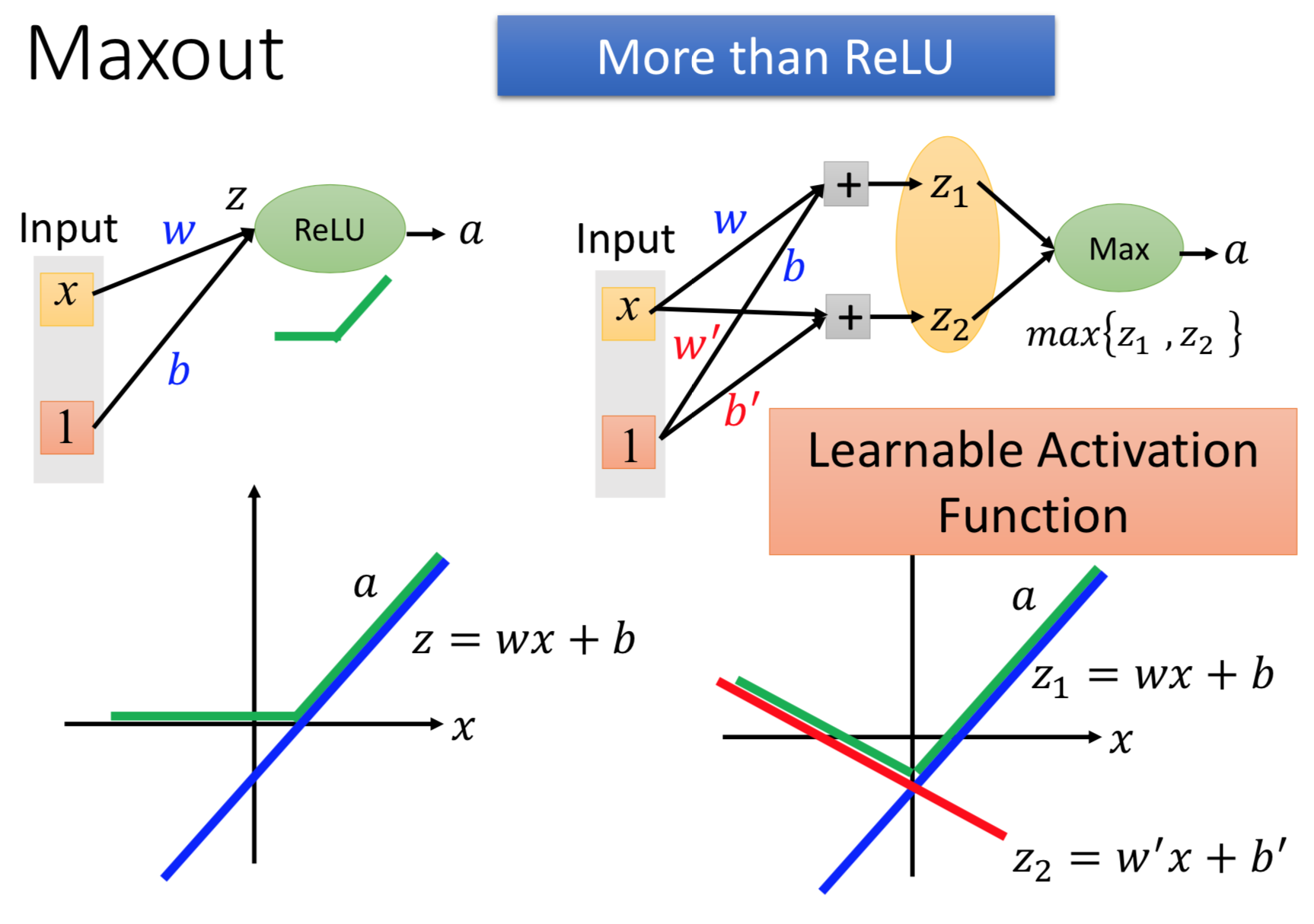$\phantom{\rule{1em}{0ex}}$$\quad$

### Maxiout方法的训练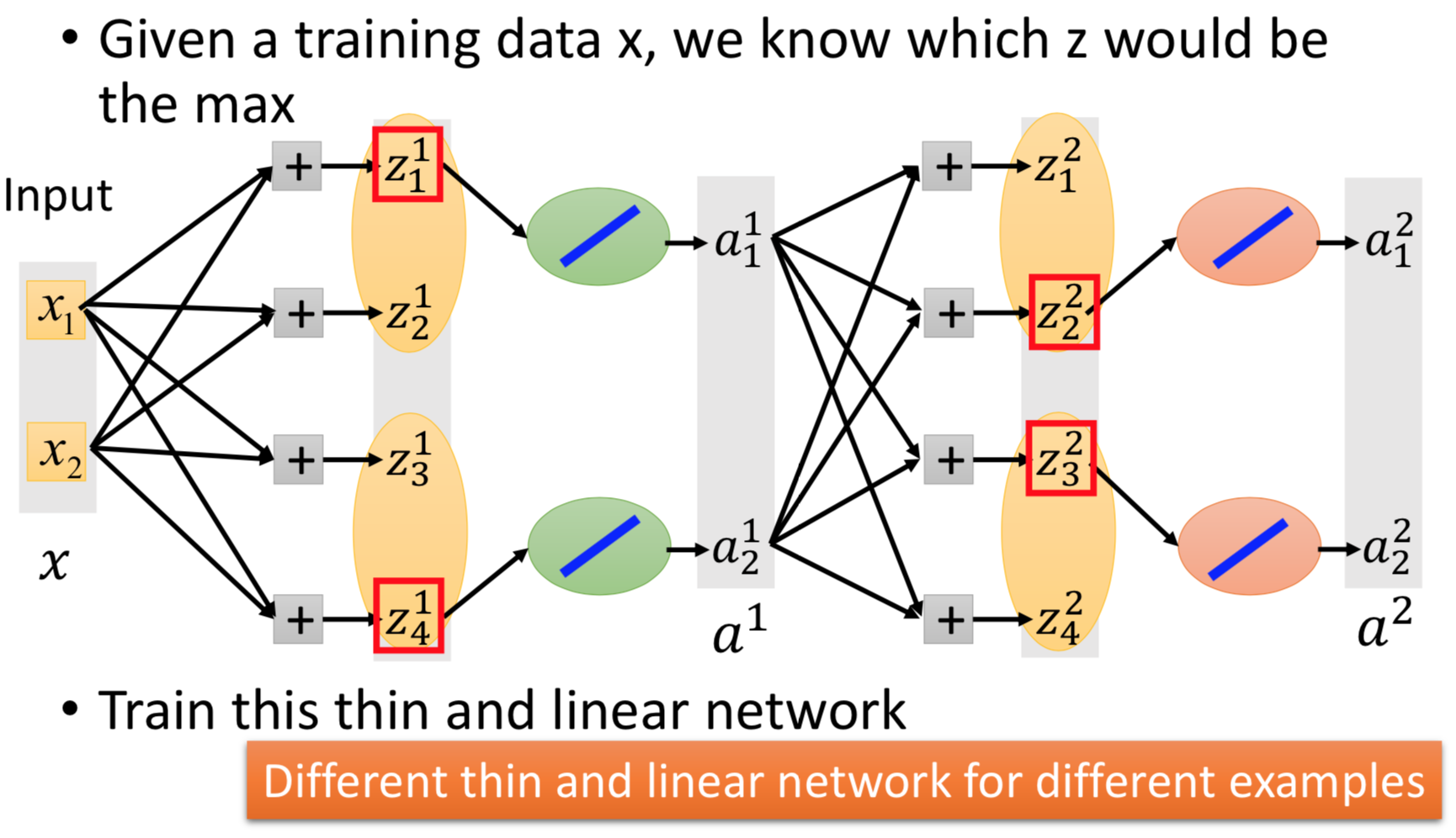$\phantom{\rule{1em}{0ex}}$$\quad$

$\phantom{\rule{1em}{0ex}}$$\quad$

## RMSProp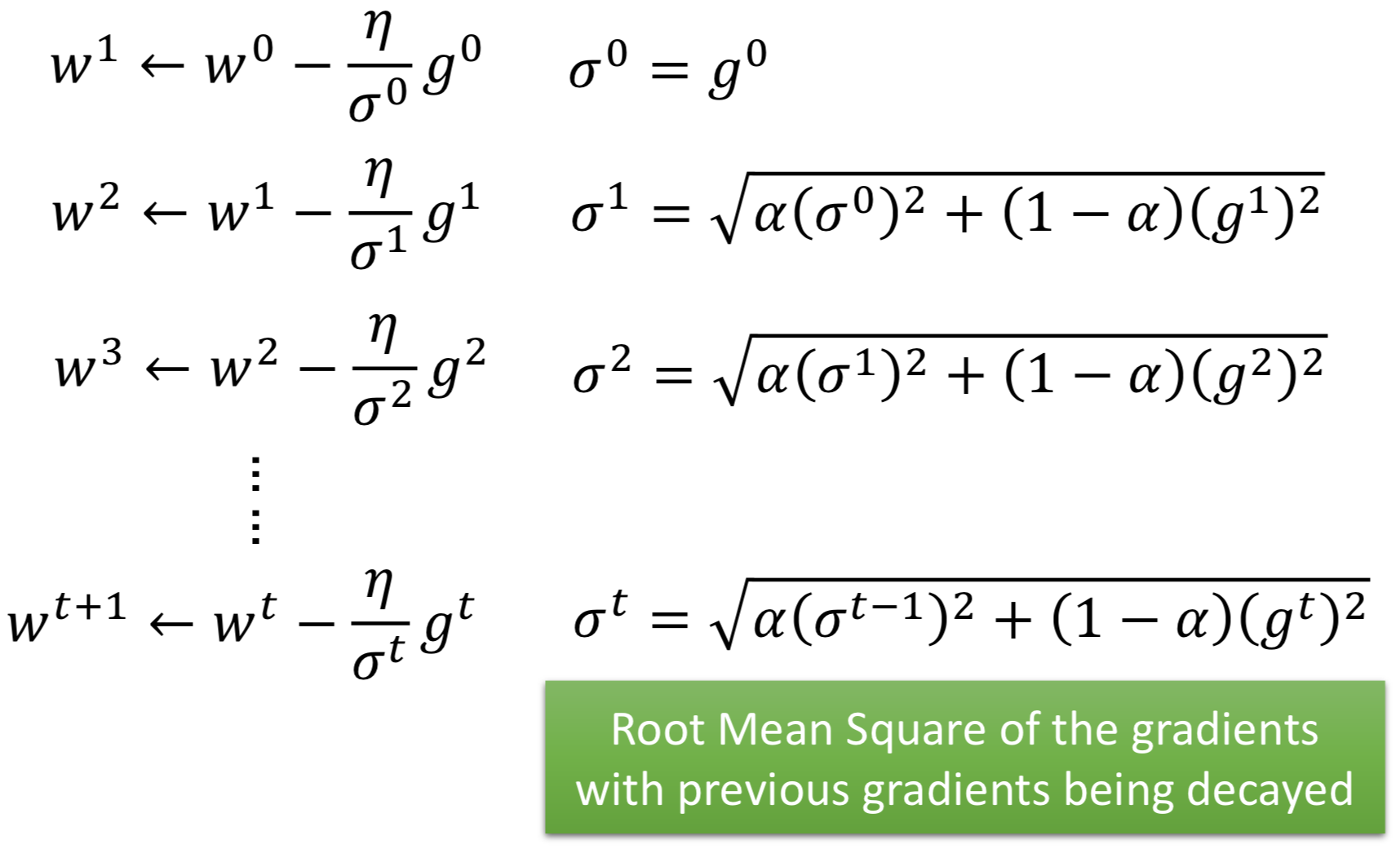$\phantom{\rule{1em}{0ex}}$$\quad$

## Momentum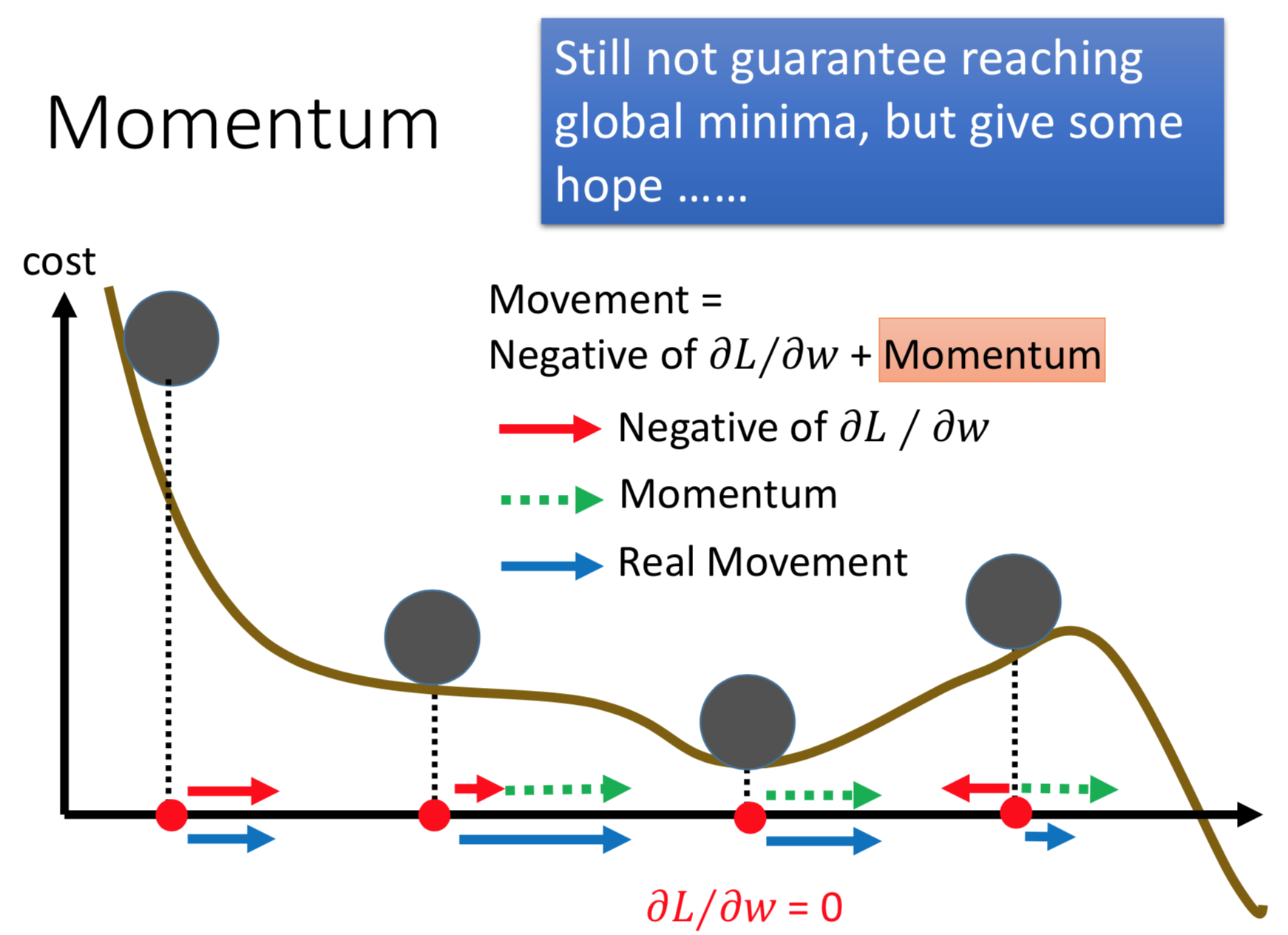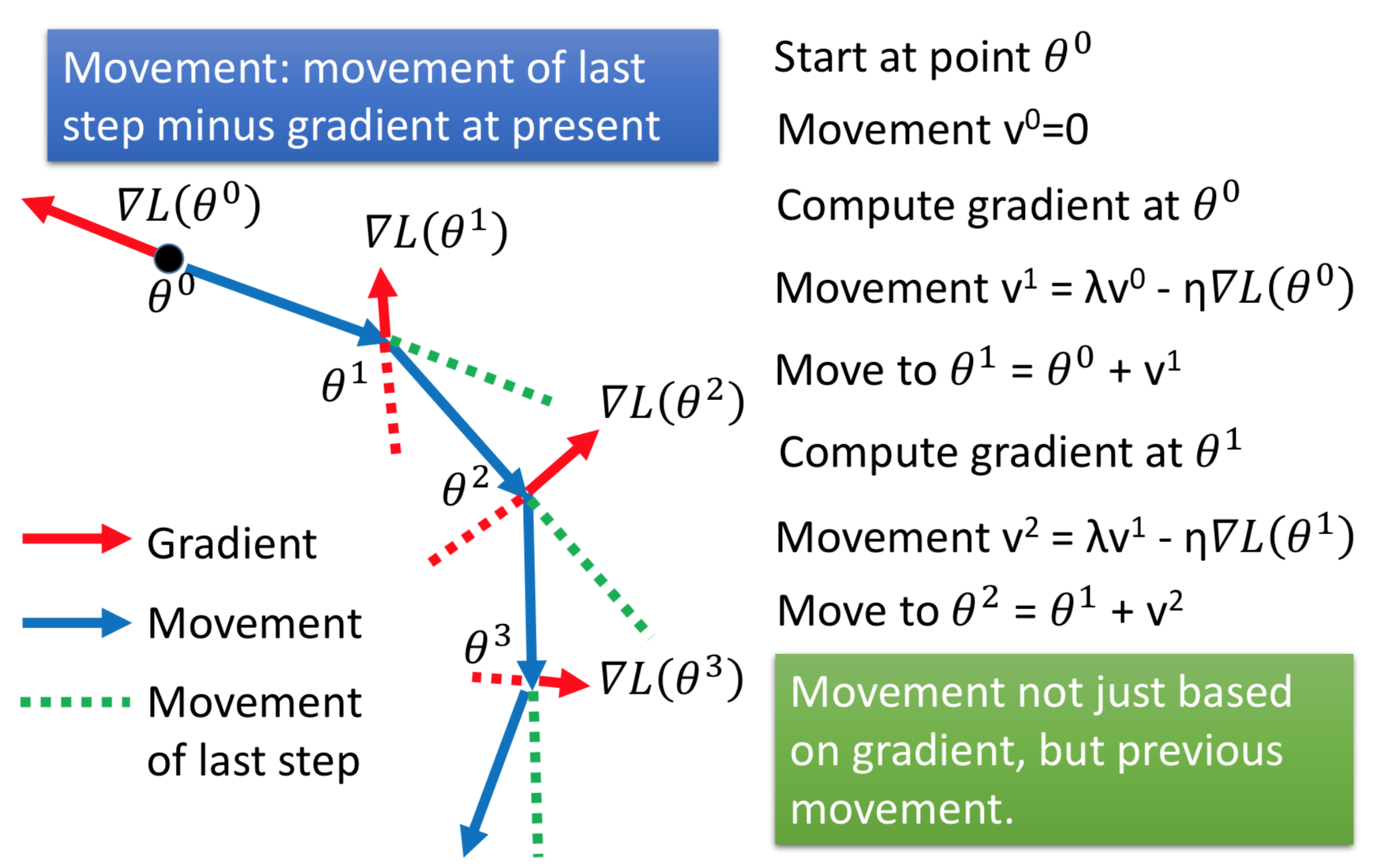$\phantom{\rule{1em}{0ex}}$$\quad$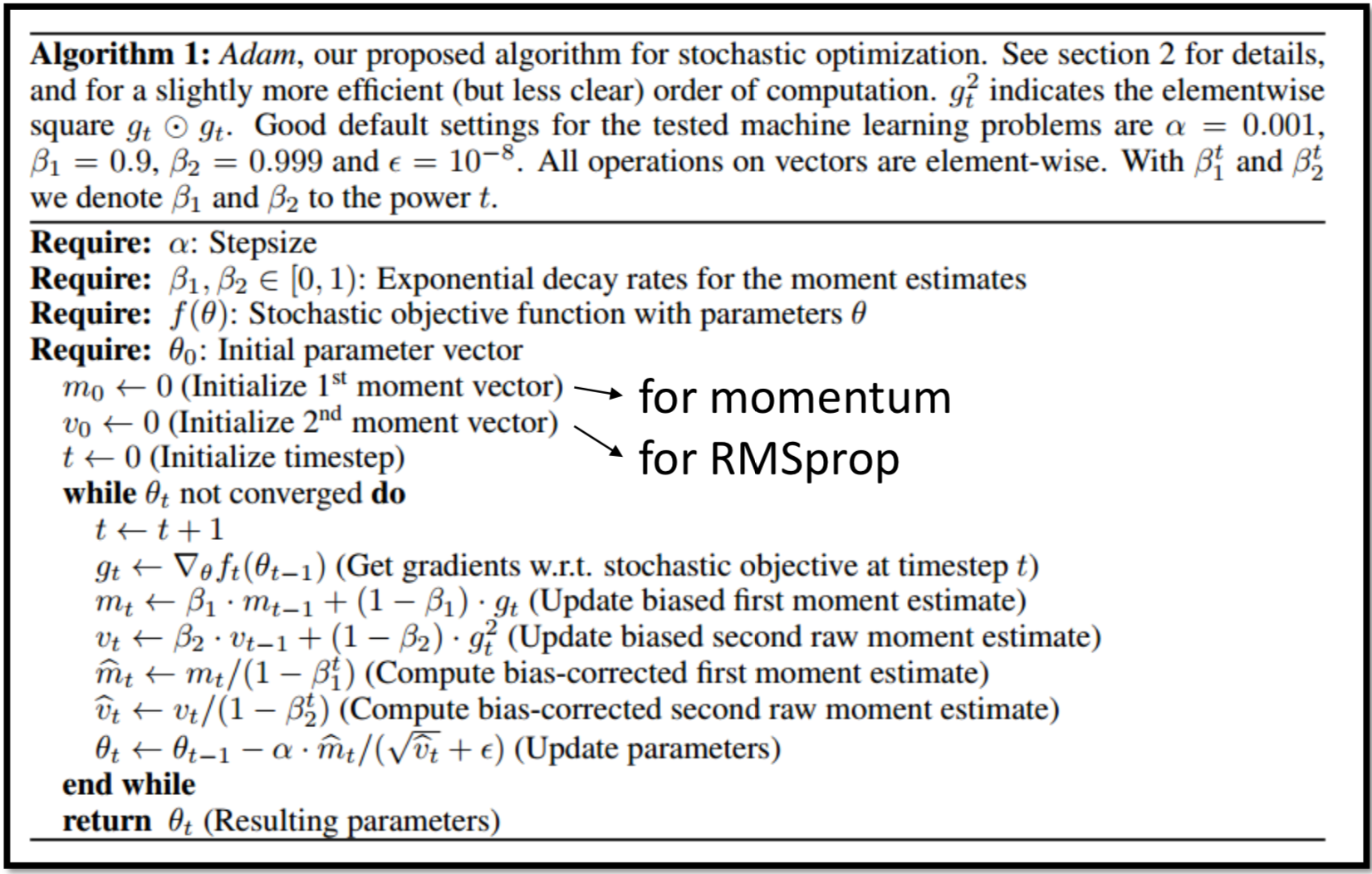$\phantom{\rule{1em}{0ex}}$$\quad$

# Early Stopping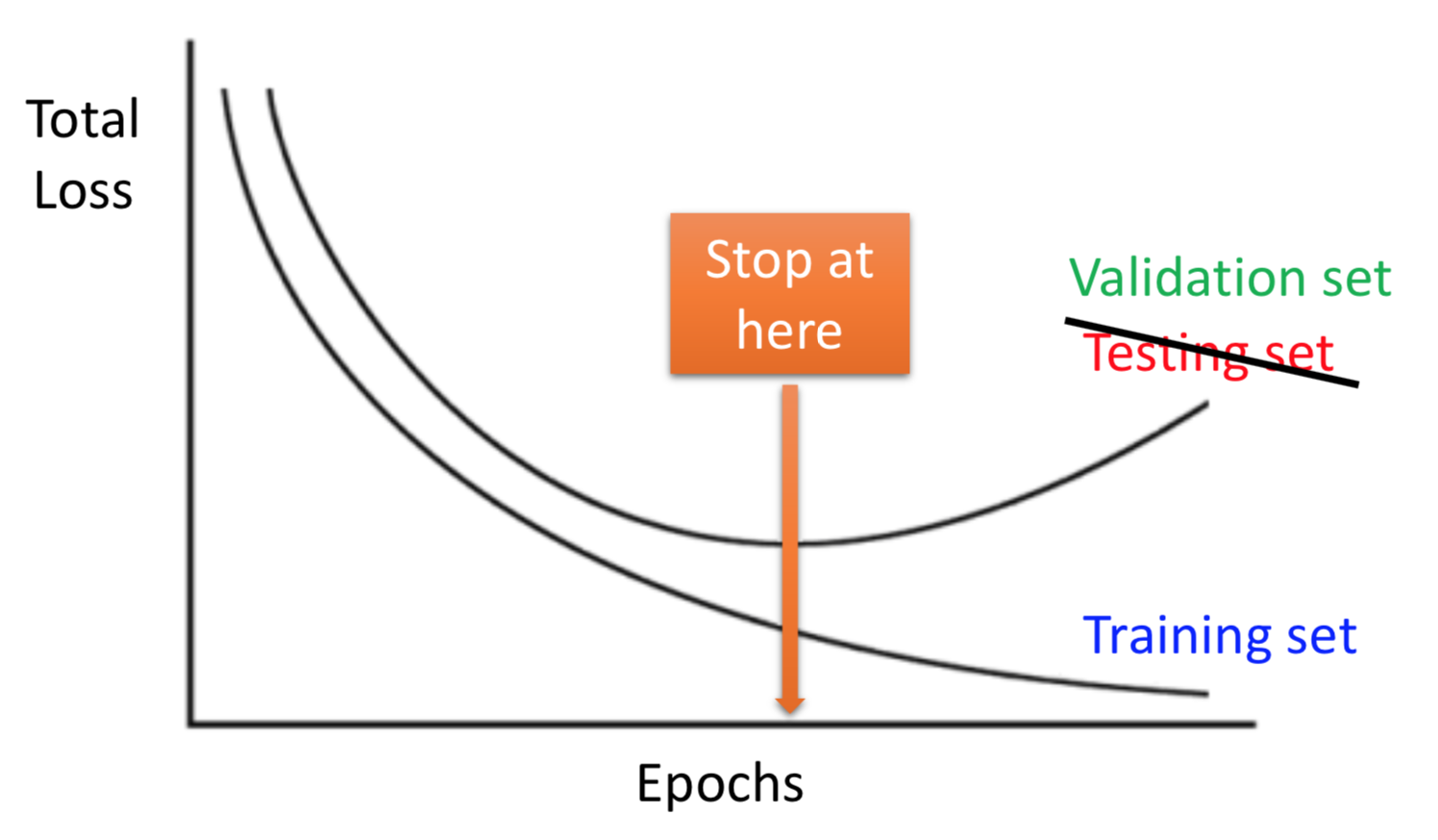$\phantom{\rule{1em}{0ex}}$$\quad$

# Regularization

## L2正则化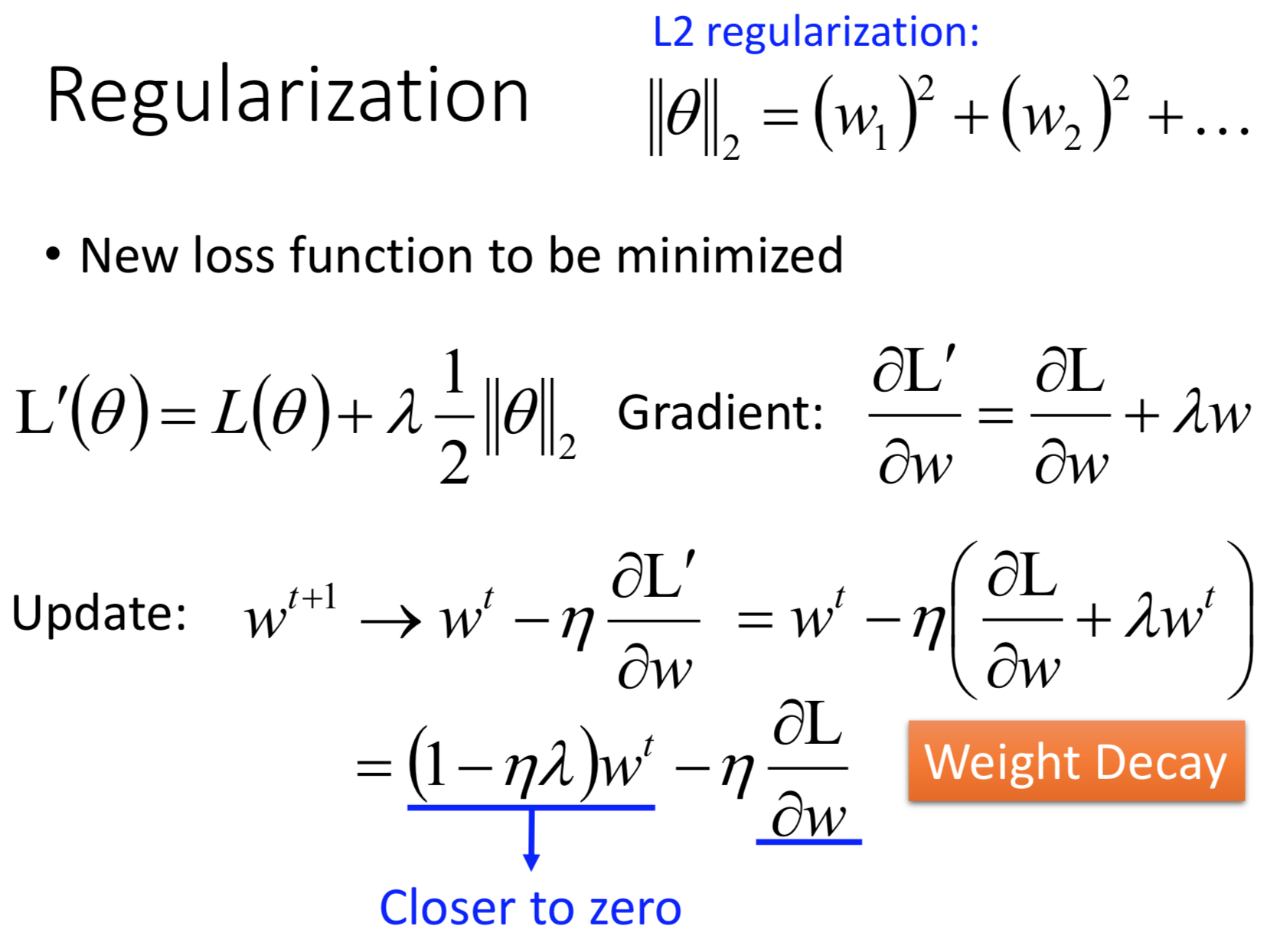$\phantom{\rule{1em}{0ex}}$$\quad$

## L1正则化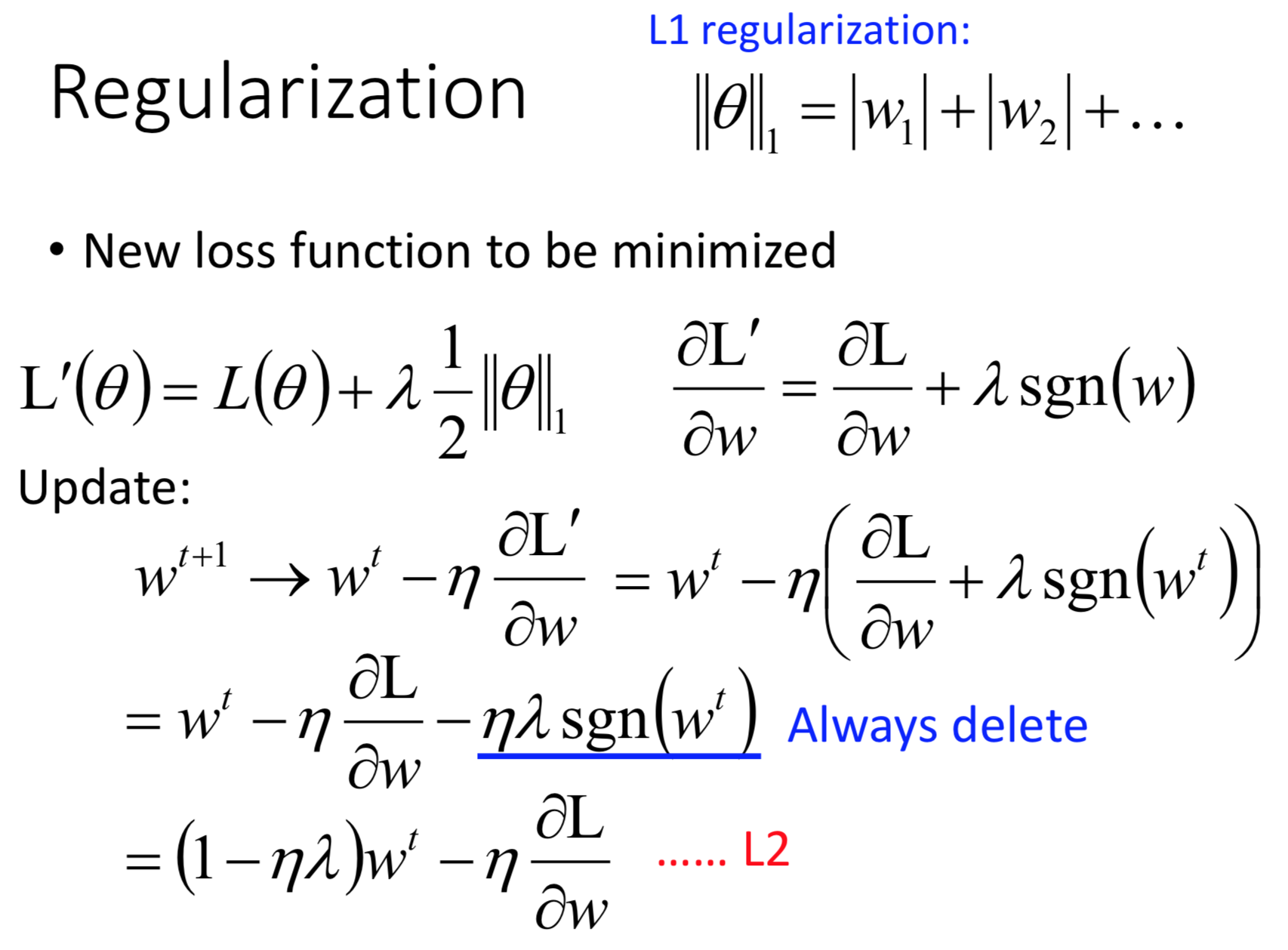$\phantom{\rule{1em}{0ex}}$$\quad$

## L2正则化vsL1正则化

L1和L2相比不同的地方在于二者都让参数接近于0，但是做法不一样：

• L2是乘以一个固定的小于1的值（$1-\eta \lambda$$1-\eta \lambda$），因此L2对比较大的参数的惩罚比较强，L2得到的参数往往聚集于0附近，但不会非常靠近
• L1是减去一个固定的值（$\eta \lambda sgn\left({w}^{t}\right)$$\eta \lambda sgn(w^t)$），L1不管参数大小，惩罚相同，得到的参数差距拉的比较大，0附近的参数更接近于0

$\phantom{\rule{1em}{0ex}}$$\quad$

# Dropout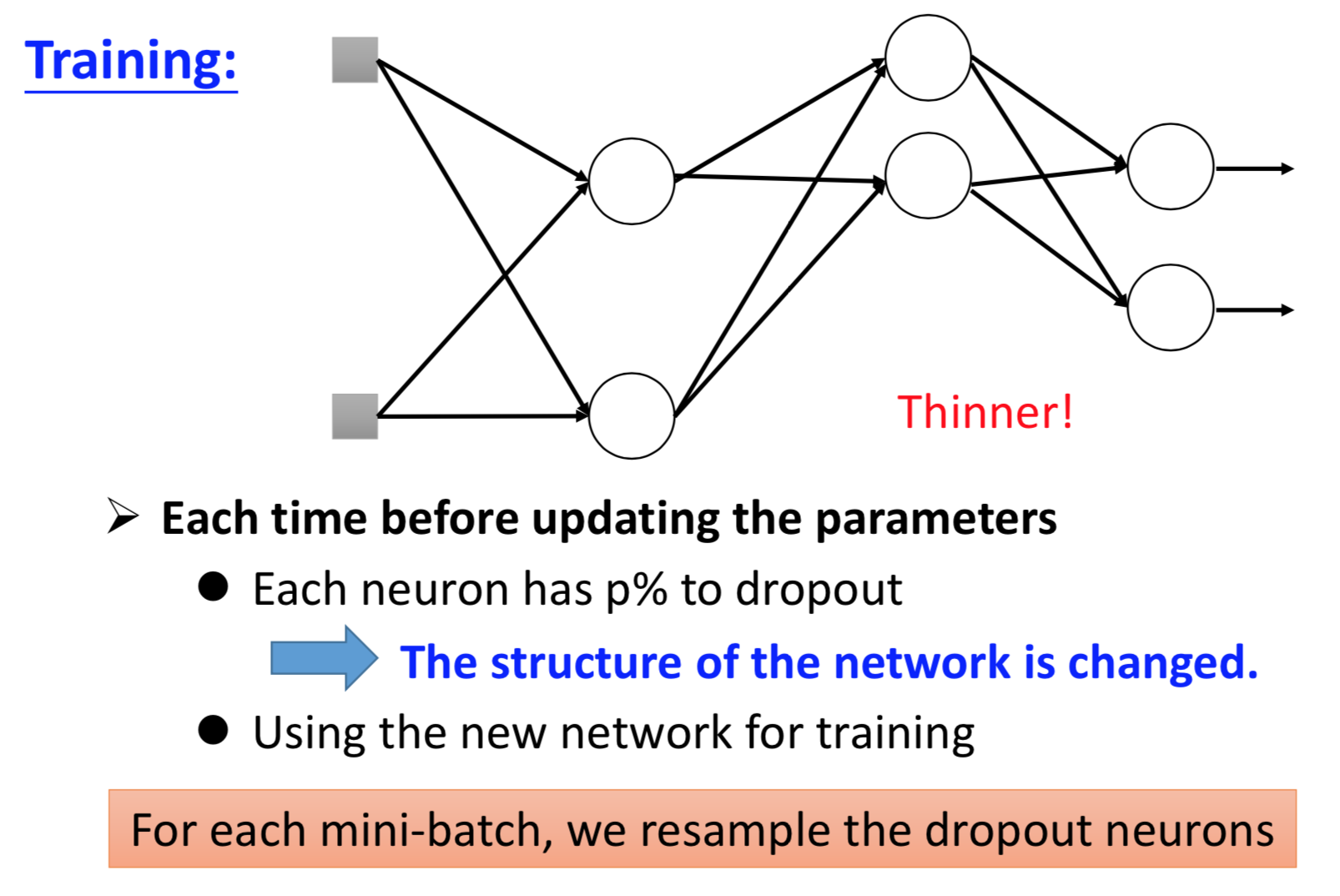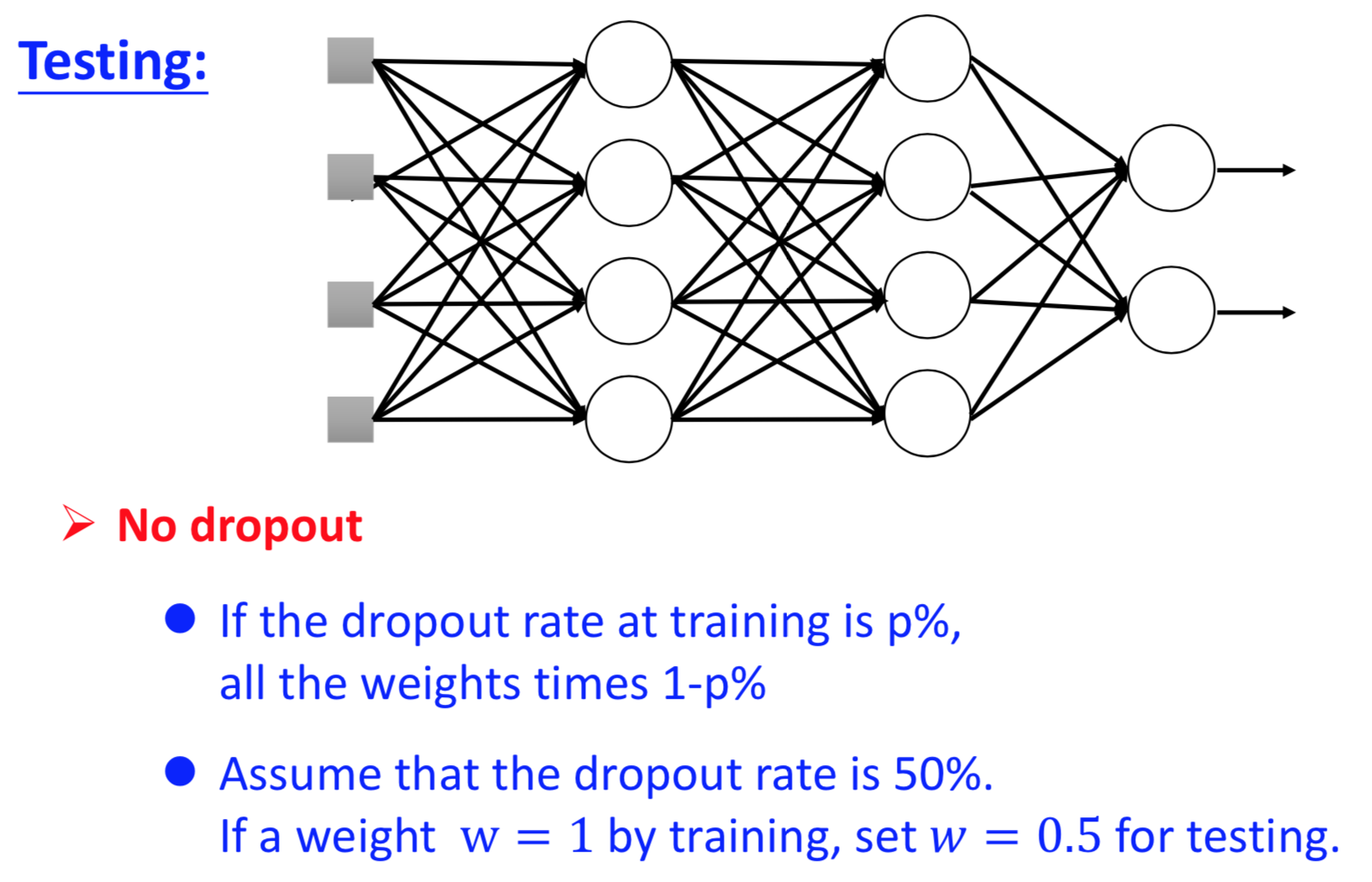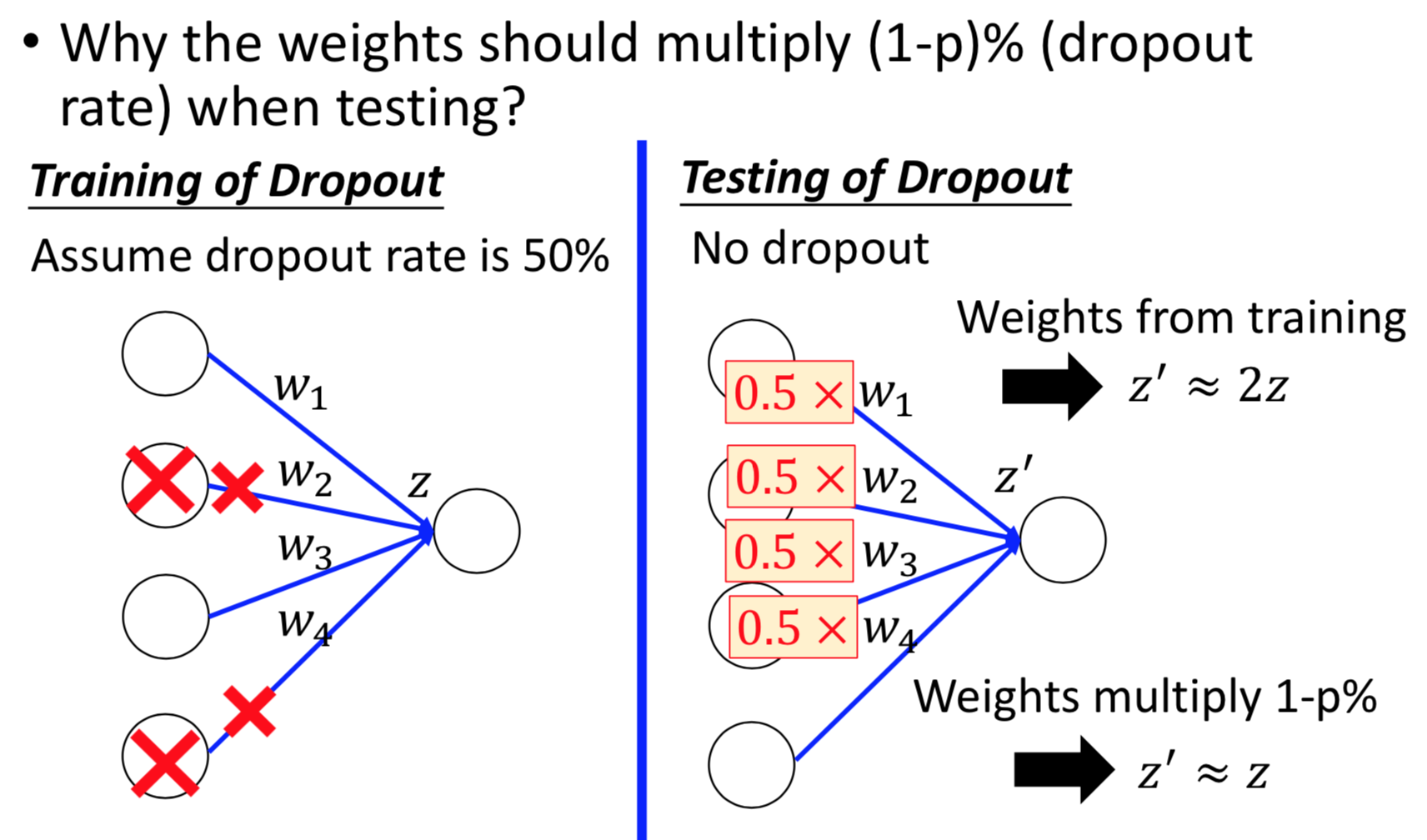Dropout本质是一种ensemble的方法，左右两个模型近似：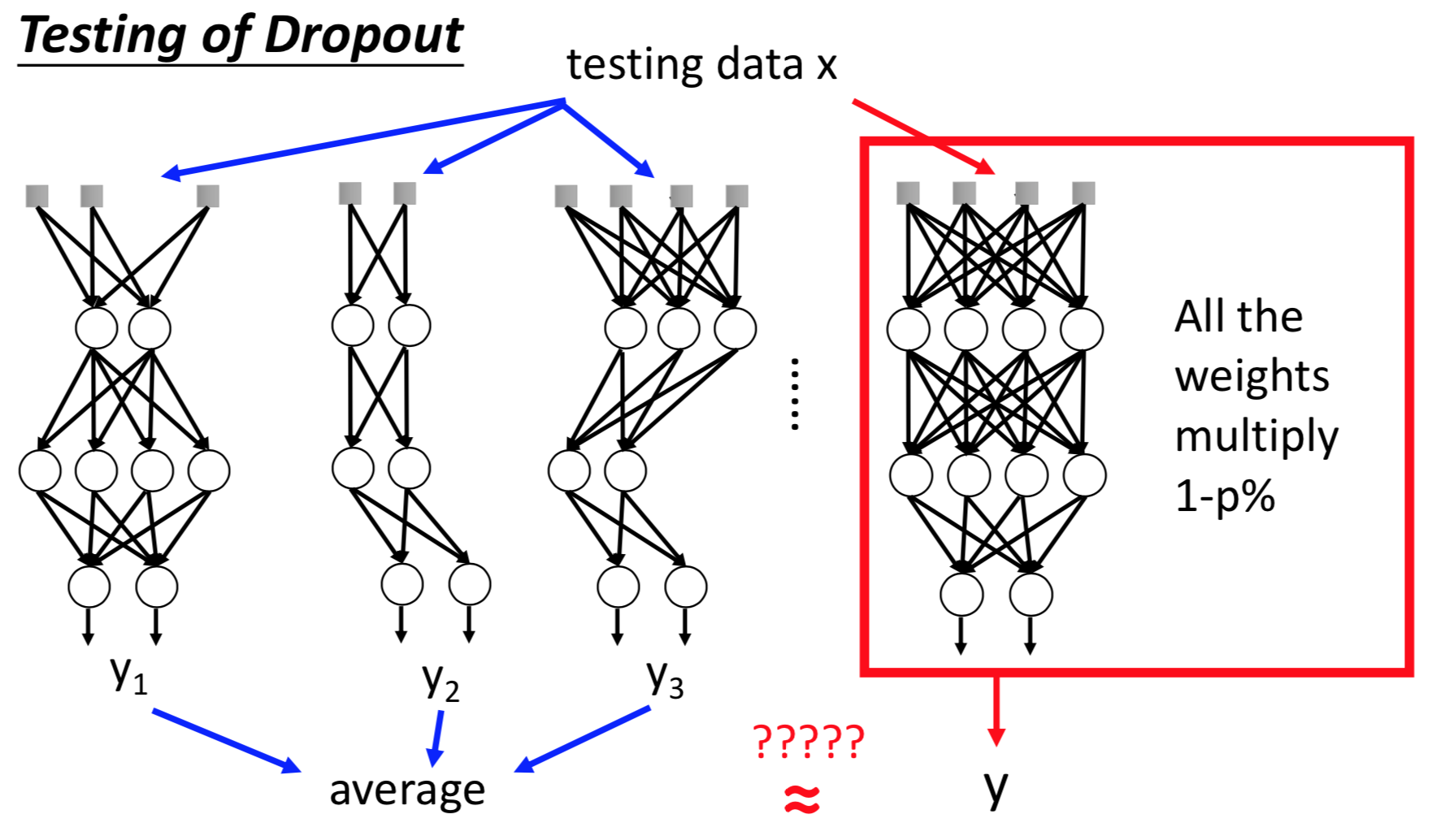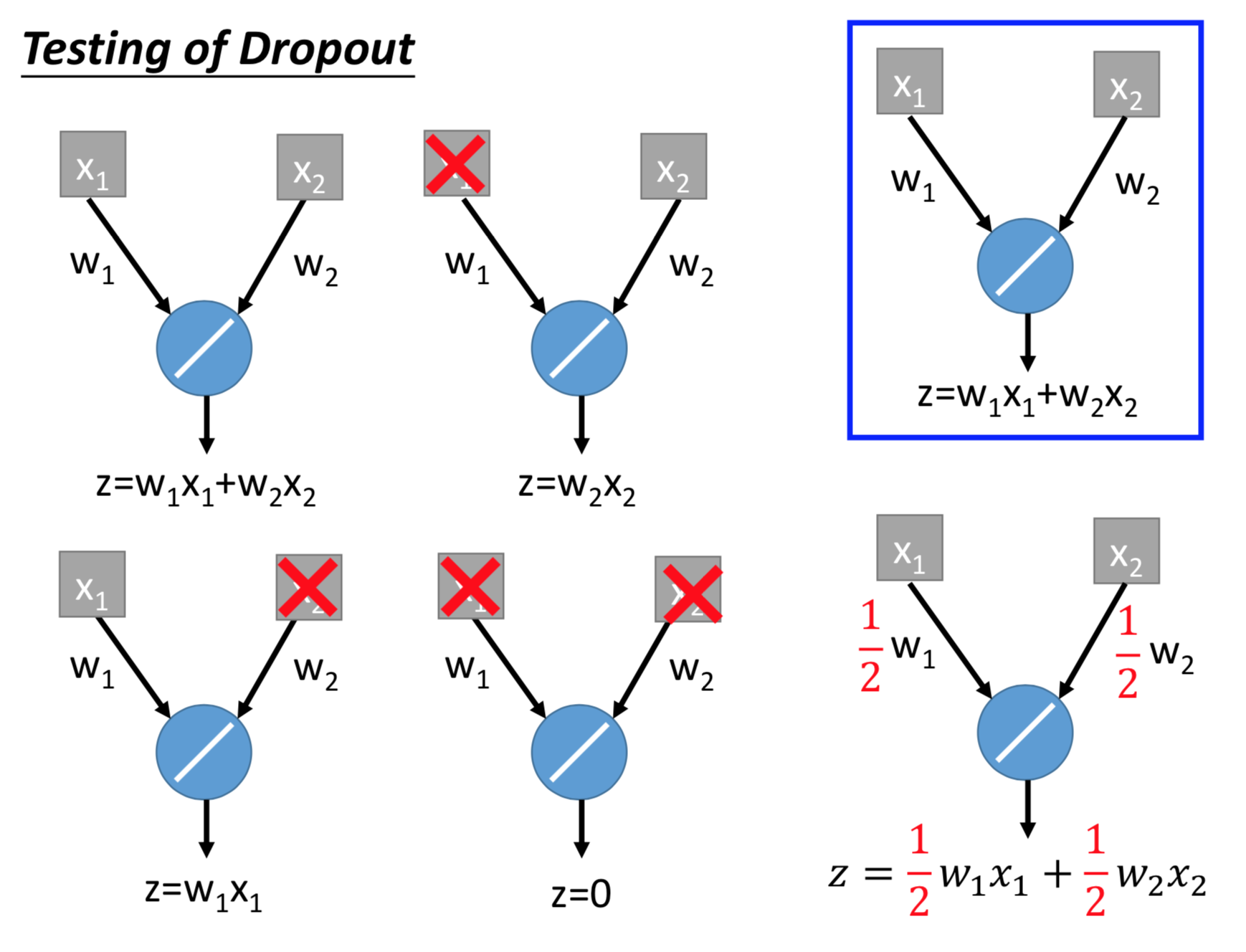2018-07-31 17:22:40 lz_peter 阅读数 853

Lecture I : Introduction of Deep Learning

• Introduction of Deep Learning
• Step1 : define a set of function
• Step2 : goodness of function
• Step3 : pick the best function
• Why Deep?
• "Hello World" for Deep Learning

Lecture II : Tips for Training Deep Neural Network

• Recipe of Deep Learning
• Choosing proper loss
• Mini-batch
• New activation function
• Momentum
• Early Stopping
• Weight Decay
• Regularization
• Dropout
• Network Structure

Lecture III : Variants of Neural Network

• Convolutional Neural Network(CNN)
• Recurrent Neural Network(RNN)

Lecture IV : Next Wave

• Supervised Learning
• Ultra Deep Network
• Attention Model
• Reinforcement Learning
• Unsuperivised Learning
• Image:Realizing what the World Looks Like
• Text:Understanding the Meaning of Words
• Audio:Learning human language without supervision